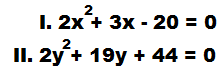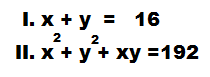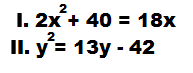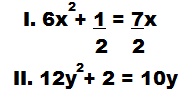# Inequalities Practice Test (2 Sets)

Today we are sharing Practice Tests of Inequalities. Try to solve these and share your marks in comment box. Keep Practicing !

### SET 1

In each of these questions, two equations (I) and (II) are given. You have to solve both the equations and given answer.

1) If x < y
2) If x > y
3) If x = y
4) If x ≥ y
5) If x ≤ y or no relationship can be established between x and y

A.B.C.
DE

A. 1

B. 4

C. 5

D. 2

E. 4

### SET-2

In each of the following questions, two equations are given. You have to solve them and give answer.

1) If x ≥ y
2) If x > y
3) If x < y
4) If x ≤ y
5) If x = y

A.
B.C.D.E.A. 3

B. 5

C. 2

D. 3

E. 4